Maslej, M., and Smardzewski, J. (2019). "Experimental testing of elastic properties of LayWood pyramidal cores," BioRes. 14(4), 9686-9703.

#### Abstract

Sandwich panels most commonly used in the furniture industry are layered structures composed of a hexagonal cell paper core. The use of wood-based composites in modelling truss and pyramidal cores of layered furniture panels is rather scarce. The effect of geometry in the auxetic truss core on the mechanical properties of manufactured wood-based materials was primarily explored in this study. Moreover, the need to conduct further studies was also stressed to determine the elastic properties of cells and cores manufactured from wood filaments using 3D printing. The aim of this study was to determine the effect of the type of filament used in 3D printing and the geometry of pyramidal core cells on elastic constants in cores with identical relative density. This paper presented analytical models of manufactured cells, results of numerical calculations performed using the finite element method, as well as experimental tests determining elastic constants of the cores. Digital image analysis was used and showed that cell geometry had a considerable effect on elastic properties of the core while maintaining identical relative density of these structures. The angle of cell arms had a particularly marked effect on these properties.

Experimental Testing of Elastic Properties of LayWood Pyramidal Cores

Michał Maslej * and Jerzy Smardzewski

Sandwich panels most commonly used in the furniture industry are layered structures composed of a hexagonal cell paper core. The use of wood-based composites in modelling truss and pyramidal cores of layered furniture panels is rather scarce. The effect of geometry in the auxetic truss core on the mechanical properties of manufactured wood-based materials was primarily explored in this study. Moreover, the need to conduct further studies was also stressed to determine the elastic properties of cells and cores manufactured from wood filaments using 3D printing. The aim of this study was to determine the effect of the type of filament used in 3D printing and the geometry of pyramidal core cells on elastic constants in cores with identical relative density. This paper presented analytical models of manufactured cells, results of numerical calculations performed using the finite element method, as well as experimental tests determining elastic constants of the cores. Digital image analysis was used and showed that cell geometry had a considerable effect on elastic properties of the core while maintaining identical relative density of these structures. The angle of cell arms had a particularly marked effect on these properties.

Keywords: Pyramidal cells; LayWood; Experiment; FEM

Contact information: Department of Furniture Design, Faculty of Wood Technology, Poznan University of Life Sciences, Poland; *Corresponding author: michal.maslej@up.poznan.pl

INTRODUCTION

The basic structural elements consisting of core and facings in layered sandwich panels are typically made from materials of high density and moduli of linear elasticity. Cores are usually composed of hexagonal, pyramidal, and truss cells or are constructed in the shell form (Wadley et al. 2003). The main materials used in their manufacture include aluminum, titanium, stainless steel (Alderson and Alderson 2007; Balawi and Abot 2008; Chen and Yan 2012), copper (He et al. 2017), polymers (Yin et al. 2013), carbon fibers (Finnegan et al. 2007; Wang et al. 2010), paper (Sam-Brew et al. 2011), and wood fibers (Smardzewski 2015). The density and mechanical properties of panels are also modified with a change in the core cell shape. For this reason designed cells need to have a comparable or identical relative density to avoid the effect of the amount of material used to construct the core on the rigidity in sandwich panels (Chen and Yan 2012; Schneider et al. 2015; Smardzewski 2015). In the furniture industry, the most commonly used sandwich panels are lightweight furniture panels comprising of a paper core with hexagonal cells. However, the results from various studies (Barboutis and Vassiliou 2005; Smardzewski and Prekrat 2012; Smardzewski 2013, 2015) have revealed that these panels are characterized by low rigidity and bending strength. To date, the research conducted has also shown that changes in geometry and dimensions of hexagonal cells of the core have a major effect on the rigidity of furniture panels (Voth and Yadama 2010; Majewski and Smardzewski 2012, 2015; Smardzewski and Prekrat 2012; Smardzewski 2013). Attempts have also been made to replace paper cores with hexagonal cells using a wavy core manufactured from wood fibers (Smardzewski and Majewski 2015; Smardzewski and Jasińska 2016), interlocking bands of plywood and high-density fiberboard (HDF) (Klimek et al. 2016), truss, and pyramidal structures typically manufactured from tin or glass or carbon fiber composites (Voth and Yadama 2010; Yin et al. 2011, 2013; Yang et al. 2015; Imbalzano et al. 2016). Wood materials have been rarely used to model truss, auxetic, and pyramidal structures. The primary aim of those attempts was to determine the elastic properties of the obtained periodic systems. In turn, numerical calculations were used to determine the effect of auxetic truss cell geometry in the core on the mechanical properties of layered wood-based panels (Wojnowska et al. 2017; Peliński and Smardzewski 2019). Those studies showed potentially advantageous elastic properties of those structures, thus suggesting their future applicability.

In contrast, few reports have considered the application of 3D printing technology using biocomposites to manufacture complex pyramidal structures in cores of layered furniture panels (Smardzewski et al. 2018). This is noteworthy, because layered panels made from wood and wood-based materials are an excellent alternative for comparable composites manufactured from metals or plastics (Xiong et al. 2010; Gao et al. 2013). They are lighter and in relation to their density they are stronger and more rigid. This facilitates efficient management of natural resources, because they are derived from renewable materials. Moreover, the applications of pyramidal structures manufactured by 3D printing from LayWood filaments are highly promising (Smardzewski and Wojciechowski 2019), and thus one of the reasons why the present study was undertaken.

The aim of this study was to determine the effect of the type of filament used in 3D printing and the geometry of pyramidal core cells on the elastic constants of the cores with identical relative density. This paper presents analytical models of manufactured cells, results of numerical calculations using the finite element method (FEM), as well as experimental results of tests for determining the elastic constants of the core.

EXPERIMENTAL

Mathematical Models

Figure 1 presents a computational model of the investigated pyramidal cell. Analysis of this geometry shows that it is composed of two perpendicular arms. The variables are as follows: Lx and Ly (mm) are the length of cells in the X and directions, respectively, lx and ly (mm) are the length of cell ribs in the X and directions, respectively, h (mm) is the cell height, and (°) are the rib angle in the ZX and ZY planes, respectively, 2a = s (mm) is the width of rib base, and t (mm) is the rib thickness. To date, the results of research conducted on similar structures have shown that the mechanical properties of panels with cell cores are considerably influenced by the length and angle of cell ribs, dimensions of rib cross-section, and the type of material from which they were manufactured, and thus also their relative density (Loinsigh et al. 2012; Gao et al. 2013; Zhang et al. 2013; Che et al. 2014; Jin et al. 2015; Zhong et al. 2015; Smardzewski et al. 2018). For this reason, it was decided to first determine the relative density of the modelled core cells.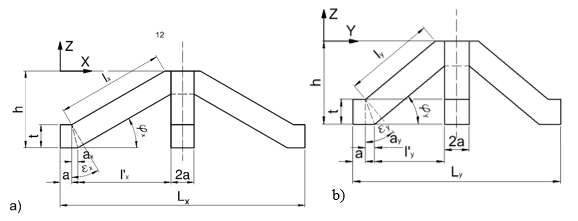Fig. 1. Elementary section of rib geometry, projection on a) ZX and b) ZY planes

Relative density (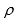) of the analyzed structure may be described as,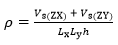(1)

where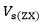(mm3) is the volume of rib in the ZX plane and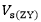(mm3) is the volume of rib in the ZY plane: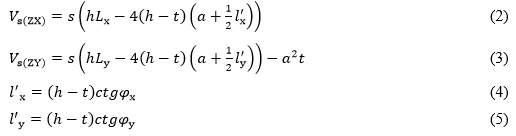In view of the varied lengths of the cell ribs (l> ly) (mm), using the diagram in Fig. 2, two moduli of linear elasticity Ex and Ey, and modulus of elasticity in shear Gxy were determined. Calculations were made applying the Maxwell-Mohr virtual force method (Wojnowska et al. 2017).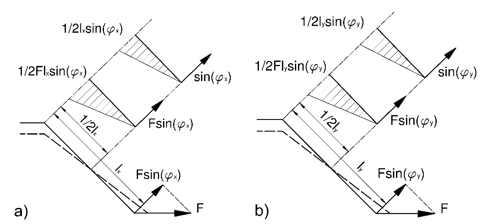Fig. 2. Diagram of loading for a rib in the elementary core section

To determine the modulus (Ex), it was assumed that normal stress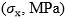in the X direction is estimated using Eq. 6,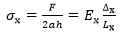(6)

where F (N) is the rib loading and Δx (mm) is the elongation caused by the action of force (F, N) (Fig. 2a). The elongation (Δx, mm) was calculated from Eq. 7,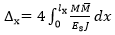(7)

where Es (MPa) is the modulus of elasticity for core substance,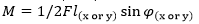(Nm) is the bending moment of rib,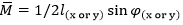(Nm) is the virtual bending moment of rib, and J (m4) is the moment of inertia of rib cross-section given by Eq. 8 as: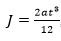, (8)

thus: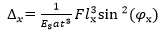(9)

By solving the system of Eq. 10: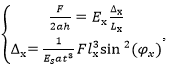, (10)

the authors obtained Eq. 11 as follows: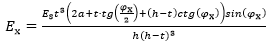(11)

When determining the modulus (Ey, MPa) it was assumed that normal stress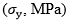in the Y direction is,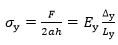(12)

where Δy (mm) is the elongation caused by the action of F (N) (Fig. 2b). Elongation (Δy, mm) was calcuated from Eq. 13 as,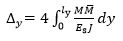(13)

thus: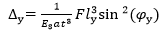(14)

By solving the system of Eq. 15,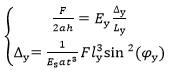(15)

the authors obtained Eq. 16 as: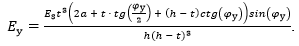. (16)

Due to the theoretical lack of transverse strain of ribs, Poisson’s ratios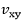and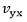take the value of zero. In turn, the equation for the shear modulus of elasticity may be presented as Eq. 17: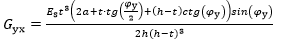(17)

Filaments

Core cells were manufactured from filaments used in 3D printing technology applying fused deposition modelling (FDM). The selected filaments containing wood dust are commercially available as 3Dbamboo (LayWood 30%, LY), 3Dwood (LayWood 40%, LB), and polyactide (PLA) (PRI-MAT 3D; Filament Prima, Tarnowo Podgórne, Poland). PLA is the most popular resin for 3D printing because it is easy to use and has almost no odor. It adheres well to masking tape without requiring a heated printing bed or noxious solvents. LayWood contains 30 % or 40 % recycled wood dust and harmless binders made by co-polyesters. Elastic properties of these materials, i.e., modulus of linear elasticity (Es), modulus of rupture (MOR, MPa), and Poisson’s ratio (v), were determined in the uniaxial tension test. For each filament, a total of 10 dumbbell samples were prepared with the shape and dimensions as in Fig. 3. Tests were conducted according to the PN-EN ISO 527-3 (1998) standard using a Zwick 1445 universal strength testing machine (Zwick GmbH, Ulm, Germany) and a Dantec dynamics optical extensimeter system (Dantec Dynamics A/S, Skovlunde, Denmark). Table 1 presents the mean values and standard deviations (in parentheses) for the elastic constants of used filaments.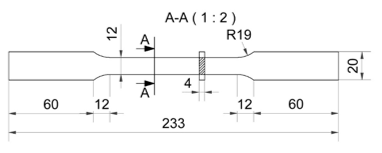Fig. 3. Dimensions of dumbbell sample for uniaxial tension tests, units in mm

Table 1. Elastic Properties of Filaments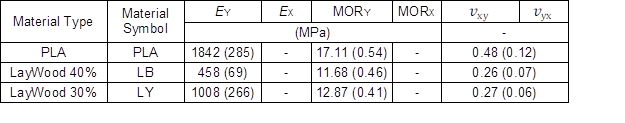Shapes of Core Cells

Based on the developed mathematical models and calculated elasticity constants for the core substance, eight types of pyramidal cells were computed, heuristically selected, and characterized by comparable relative density, but differed in shape and dimensions (Fig. 4). Cell A is a reference object, characterized by rib angle . Table 2 presents the most important dimensions and values of elastic constants calculated for the selected cells. At this stage, it was assumed that cells were manufactured from PLA. In the next step, cell shape was optimized using the Monte Carlo method, consisting in random search through the admissible area, and on the basis of obtained results the optimal value was estimated. The aim of that step was to produce cells noticeably differing from those heuristically identified and exhibiting extreme properties. The mathematical model for the optimization of rib cells included:

Decision variables, for which the block of variables Kz takes the form,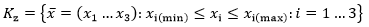(18)

where i is the the number of decision variables for cells, xt = 2a (mm),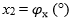is the angle of cell ribs in the ZX plane, and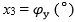is the angle of cell walls in the ZY plane:

1. Parameter Es constituting the modulus of linear elasticity (MPa) for the material, from which ribs were manufactured, and
2. Set of admissible values Φ is composed of inequality bounds Φi(x)>0i.e.: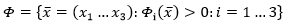(19)

The objective function was assumed to consist in the minimization of relative density of cells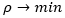as well as maximization of values of moduli of linear elasticity of cells in the main orthotropic directions: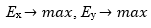(20)

When attempting at optimization, it is necessary to provide minimal, intuitively estimated values of cell dimensions tmin (mm),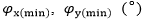and maximal dimensions tmax (mm),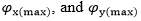(°), which are to be optimized. In the block of decision variables these values are described by extreme nodes Dmin and Dmax. During the optimization process, the computer randomly selected from a given block any nodes D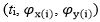and remembered only those of them, which met all the limiting conditions. The node best describing the objective function represents optimal cell dimensions. A similar Monte-Carlo optimization algorithm was presented in Peliński and Smardzewski (2019).

The input data for the Monte-Carlo optimization function were comprised of the following values: tmin = 2.0 mm, tmax = 5.0 mm,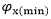= 25°,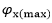= 65°,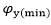= 25°,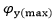= 65°, and Es (MPa) as given in Table 1 (for LayWood 40%). Adopting the number of sampling N= 30000, the optimization process was completed, as the result of samplings was not improved. On this basis, four additional cells were selected, as presented in Fig. 5, in which the representative numerical values are given in Table 2.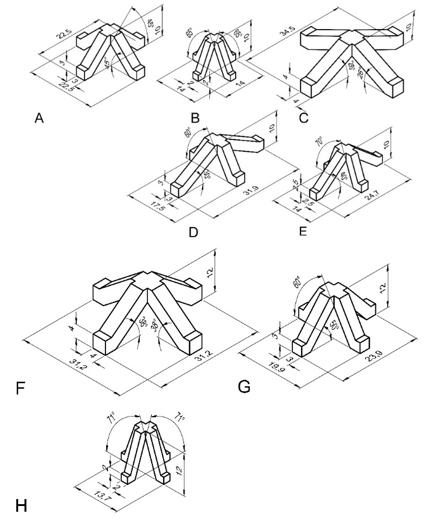Fig. 4. Geometry of selected core cells: For models A to E h = 10 mm and for models F to H h = 12 mm, all units in mm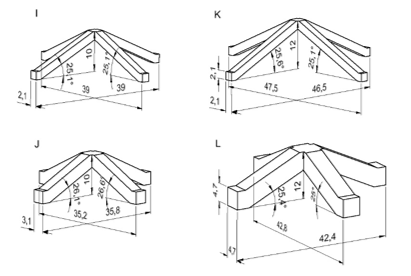Fig. 5. Geometry of optimized cells: For models I to J h =10 mm and for models K to L h = 12 mm, all units in mm

Table 2. Characteristic Properties of Selected Pyramidal Cells. LayWood 40% Filament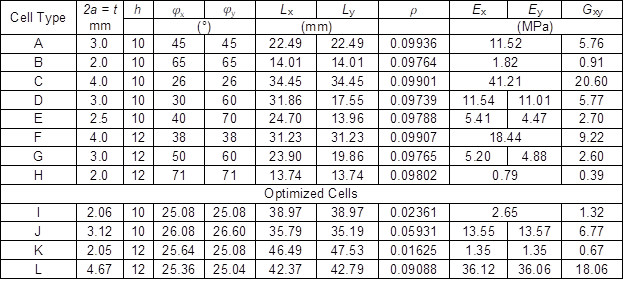Numerical Models

To verify the quality of the analytical models, FEM computations were performed for pre-selected pyramidal cores, as presented in Table 2. In view of the diversity in cell-arm size, it was assumed that each core should contain at least three cells in both directions, X and Y. Thus, the formed models were used in the numerical analysis by the FEM analysis. An example of a computer model for a pyramidal core with cell A is presented in Fig. 6.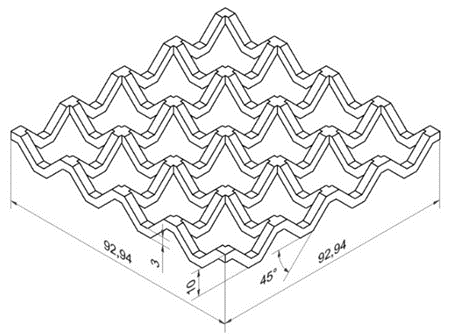Fig. 6. A CAD (computer aided design) model for rib core type A, all units in mm

Calculations were performed using the Abaqus v.6.13 program (Dassault Systemes Simulia Corp., Waltham, MA, USA) and the EAGLE computing cluster (Poznań Supercomputing and Networking Center, PSNC, Poznan, Poland) within the computing grant “Properties of furniture panels with synclastic face and auxetic core”. The computing model is presented in Fig. 7. The tetrahedral mesh was applied along with elastic-plastic strains for selected materials. The models had a mean number of nodes at 0.5 to 1.2 million and a mean number of elements at 0.3 to 1 million. Cubic elements C3D6 and C3D8R were used.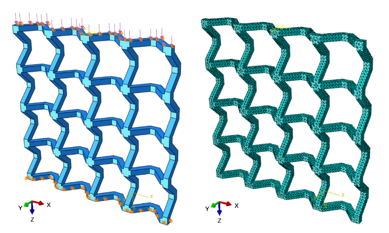Fig. 7. An example model of the core used in numerical calculations

Computations were performed for loads in the direction of the X-axis and next the Y-axis, recording the respective elongations Δx and Δy (mm). Appropriate compressive loads were adopted at values from 0 N to 15 N at every 3 N for cells type G and H, from 0 N to 30 N at every 5 N for cells type B, I, J, K, and L, from 0 N to 60 N at every 10 N for cells type E and F, and from 0 N to 120 N at every 20 N for cells type A, C, and D. Loads were selected depending on rib thickness and their angle. The values of elastic constants obtained from numerical calculations are given in Table 3.

Experiment

It was decided to experimentally verify the results of analytical and numerical computations. For the purpose of this experiment, physical models of the core were constructed using 12 different pre-selected cell geometries. Three types of previously described filament types were applied in 3D printing. For each geometry type, five samples were prepared, providing a total of 180 samples (Figs. 8 and 9).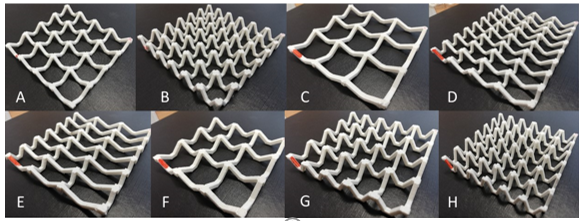Fig. 8. Models of pyramidal cell cores A to H